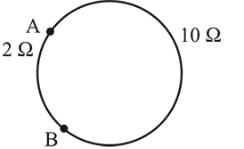# A metal wire of resistance

Question:

A metal wire of resistance $3 \Omega$ is elongated to make a uniform wire of double its previous length. This new wire is now bent and the ends joined to make a circle. If two points on the circle make an angle $60^{\circ}$ at the centre, the equivalent resistance between these two points will be:

1. (1) $\frac{12}{5} \Omega$

2. (2) $\frac{5}{2} \Omega$

3. (3) $\frac{5}{3} \Omega$

4. (4) $\frac{7}{2} \Omega$

Correct Option: , 3

Solution:

(3) When length becomes double its resistance becomes

$\left(R \propto l^{2}\right)$

$R=4 \times 3=12 \Omega$

$R_{\mathrm{eq}}=\frac{2 \times 10}{12}=\frac{5}{3} \Omega$